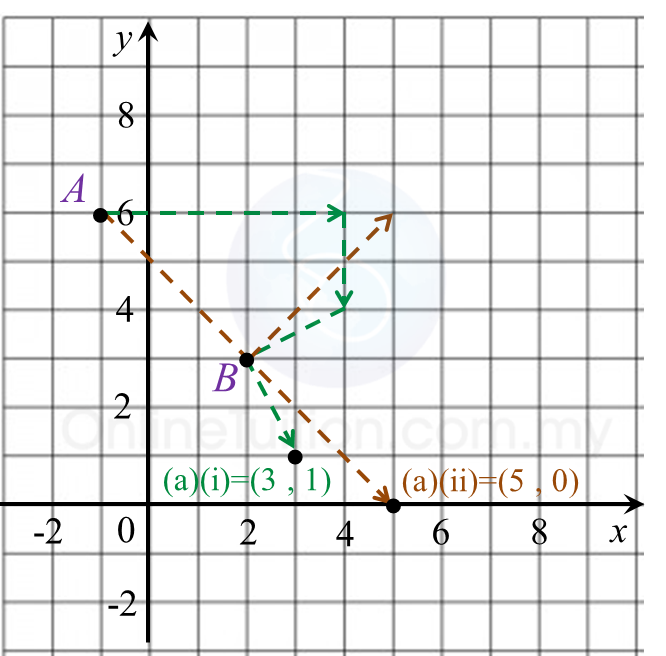# 3.3.4 Transformation III, SPM Paper 2 (Long Questions)

Question 7:
Diagram shows the point J(1, 2) and quadrilaterals ABCD and EFGH, drawn on a Cartesian plane.(a)
Transformation U is a rotation of 90o, clockwise about the centre O.
Transformation T is a translation $\left(\begin{array}{l}\text{2}\\ 3\end{array}\right)$
Transformation R is a reflection at the line x = 3.

State the coordinates of the image of point J under each of the following transformations:
(i) RU,
(ii) TR.

(b)
EFGH is the image of ABCD under the combined transformation MN.
Describe in full, the transformation:
(i) N,
(ii) M.

(c)
It is given that quadrilateral ABCD represents a region of area 18 m2.
Calculate the area, in m2, of the shaded region.

Solution:(a)
(i) J (1, 2) → U → (2, –1 ) → R → (4, –1)
(ii) J (1, 2) → R → (5, 2) → T → (7, 5)

(b)(i)
N is a reflection in the line x = 6.

(b)(ii)
M is enlargement of scale factor 3 with centre (8, 7).

(c)
Area EFGH = (scale factor)2 x Area of object ABCD
= 32 x 18
= 162 m2

Therefore,
= Area of EFGH – Area of ABCD
= 162 – 18
= 144 m2

Question 8:
(a) Diagram 8.1 shows point A and point B marked on a Cartesian plane.Diagram 8.1

Transformation R is a rotation of 90o, clockwise about the centre B.

Transformation T is a translation

State the coordinates of the image of point A under each of the following transformations:
(i) RT,
(ii) R2.

(b)
Diagram 8.2 shows three trapeziums ABCD, PQRS and TUVS, drawn on a Cartesian plane.Diagram 8.2

(i)
Trapezium PQRS is the image of trapezium ABCD under the combined transformation MN.
Describe in full, the transformation:
(a) N,
(b) M.

(ii)
It is given that trapezium ABCD represents a region of area 30 m2.
Calculate the area, in m2, of the shaded region.

Solution:
(a)(i)
A (–1, 6) → T → (4, 4 ) → R → (3, 1)
(ii) A (–1, 6) → R → (5, 6) → R → (5, 0)

(b)(i)(a)
N is a reflection in the line y = 4.

(b)(ii)(b)
is enlargement of scale factor 2 with centre S (1, 5).

(b)(ii)
Area of PQRS = (scale factor)2 x Area of object ABCD
= 22 x 30
= 120 m2

Therefore,